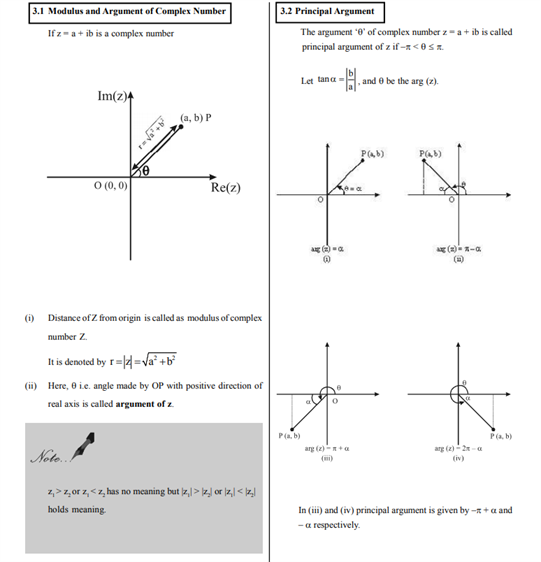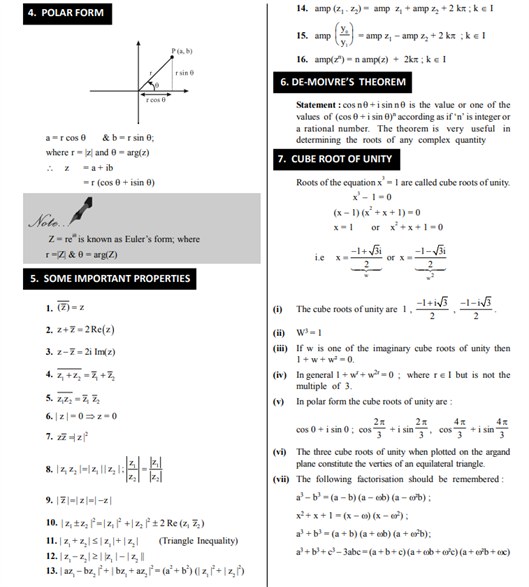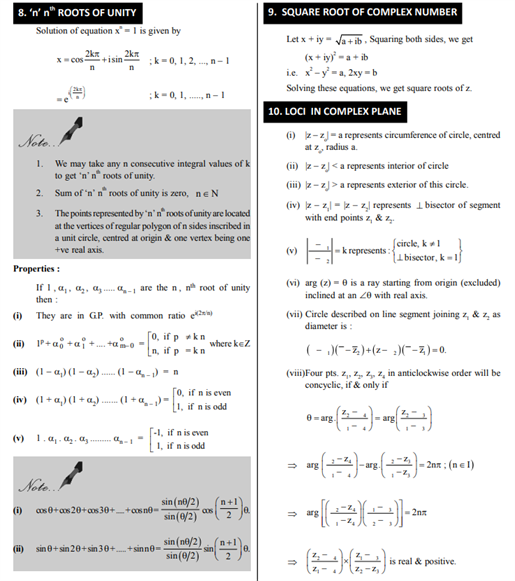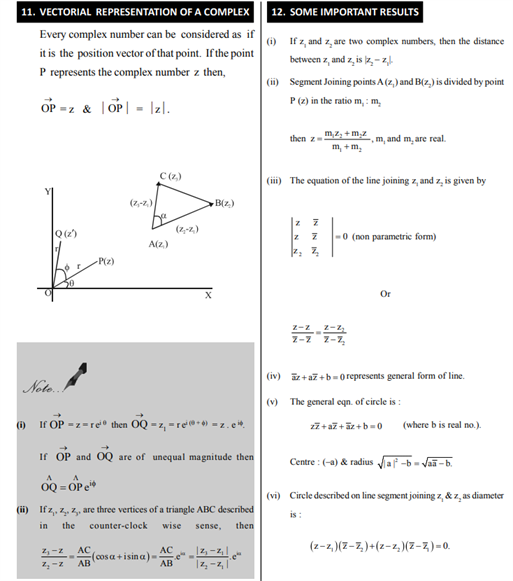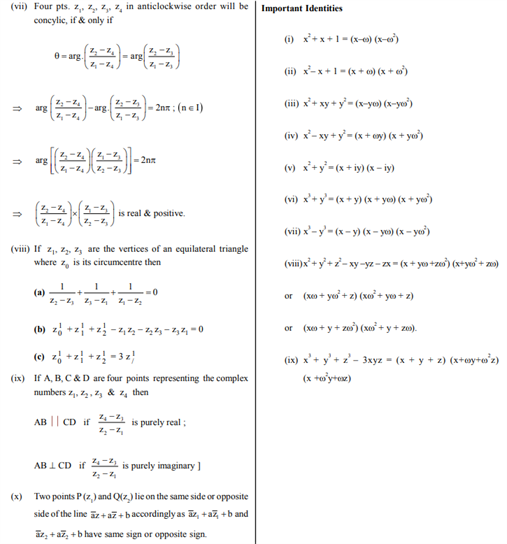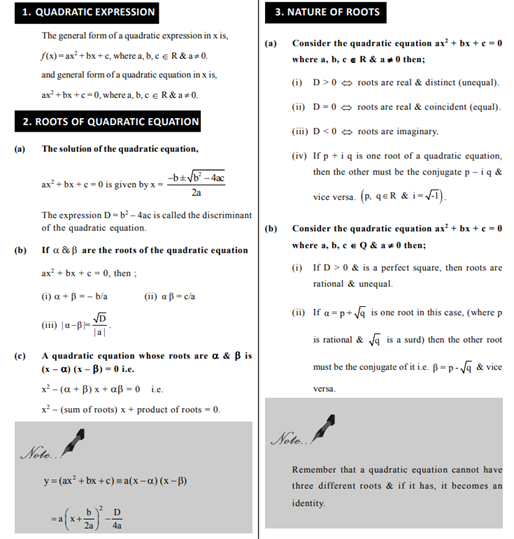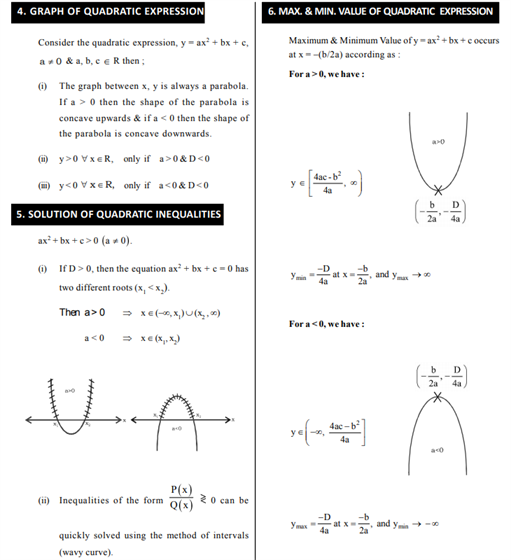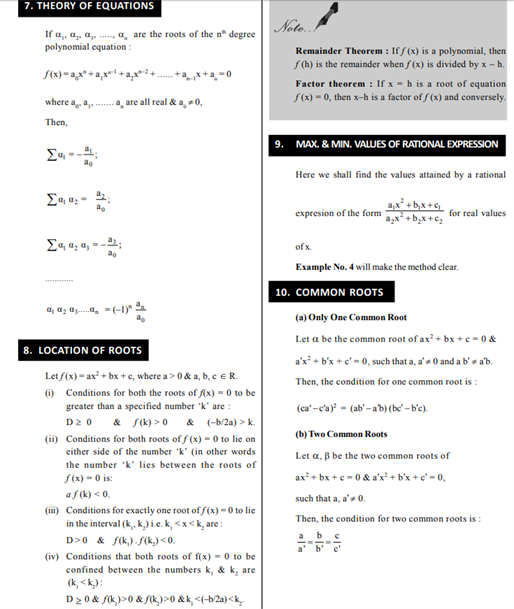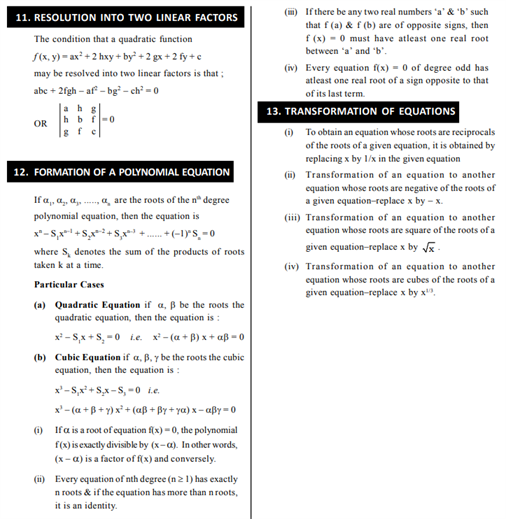# Revision Notes For CBSE Class 11 Maths Chapter 5 Complex Number And Quadratic Equations

Class 11 Maths Revision Notes Chapter 5 Complex Numbers and Quadratic Equations brings a compilation of all the important concepts to learn in this chapter. These notes are designed carefully by subject experts who have explained in detail the need to know facts about topics in every chapter. The definition of a complex number and quadratic equation are:

• A complex number can be written in the form a + bi where a and b are real numbers (including 0) and i is an imaginary number.

Therefore a complex number contains two ‘parts’: one that is real and another part that

is imaginary

Note: Even though complex has an imaginary part, there are actually many real life applications of these “imaginary” numbers

• A quadratic equation is an equation of the second degree, meaning it contains at least one term that is squared. The standard form is ax² + bx + c = 0 with a, b, and c being constants, or numerical coefficients, and x is an unknown variable. One absolute rule is that the first constant “a” cannot be a zero

Students can find various explanations of concepts like argand plane and algebra of complex numbers. Students can find the definition of terms such as De-Moivre’s theorem, quadratic expression etc and along with that, they will be seeing tables mentioning the roots of equations. These topics, if prepared with the help of these notes, will yield fruitful results.

Check out the class 11 revision notes for maths Chapter 5 Complex Numbers and Quadratic Equations